Home Practice
For learners and parents For teachers and schools
Textbooks
Full catalogue
Pricing SupportLog in

We think you are located in United States. Is this correct?

# End of chapter exercises

## End of chapter exercises

Textbook Exercise 6.14

Write the following as a single trigonometric ratio: $$\dfrac{\cos (\text{90}\text{°} - A) \sin \text{20}\text{°}}{\sin (\text{180}\text{°} - A) \cos \text{70}\text{°}} + \cos (\text{180}\text{°} + A) \sin (\text{90}\text{°} + A)$$

\begin{align*} & \frac{\sin A \cdot \sin \text{20}\text{°}}{\sin A \cdot \sin( \text{90}\text{°} - \text{70}\text{°})} + (-\cos A)\cos (A) \\ &= \frac{\sin A \cdot \sin \text{20}\text{°}}{\sin A \cdot \sin \text{20}\text{°}} + (-\cos A)\cos A\\ &= 1 - \cos^2 A\\ &= \sin^2 A \end{align*}

Determine the value of the following expression without using a calculator: $$\sin\text{240}\text{°} \cos\text{210}\text{°} - \tan^2 \text{225}\text{°} \cos\text{300}\text{°} \cos\text{180}\text{°}$$

\begin{align*} & \sin(\text{180}\text{°} + \text{60}\text{°}) \cdot \cos(\text{180}\text{°} + \text{30}\text{°}) - \tan^2(\text{180}\text{°} + \text{45}\text{°}) \cdot \cos(\text{360}\text{°} - \text{60}\text{°}) \cdot (-1)\\ &= (-\sin \text{60}\text{°}) \cdot (-\cos \text{30}\text{°}) - (\tan^2 \text{45}\text{°}) \cdot (\cos \text{60}\text{°} ) \cdot (-1) \\ &= + \frac{\sqrt{3}}{2} \cdot \frac{\sqrt{3}}{2} - (1)^2 \cdot (\frac{1}{2}) \cdot (-1) \\ &= \frac{3}{4} + \frac{1}{2} \\ &= \frac{3 + 2}{4} \\ &= 1 \frac{1}{4} \end{align*}

Simplify: $$\dfrac{\sin (\text{180}\text{°} + \theta) \sin (\theta + \text{360}\text{°})}{\sin (-\theta) \tan (\theta - \text{360}\text{°})}$$

\begin{align*} &\frac{\sin(\text{180}\text{°} + \theta) \cdot \sin(\theta + \text{360}\text{°})}{\sin(-\theta) \cdot \tan(\theta - \text{360}\text{°})}\\ &= \frac{(- \sin \theta) \cdot \sin \theta}{(-\sin \theta) \cdot (- \tan(\text{360}\text{°} - \theta))}\\ &= \frac{\sin \theta}{\tan \theta} = \frac{\sin \theta}{1} \cdot \frac{\cos \theta}{\sin \theta}\\ &= \cos \theta \end{align*}

Without the use of a calculator, evaluate: $$\dfrac{3\sin \text{55}\text{°} \sin^2 \text{325}\text{°}}{\cos (-\text{145}\text{°})} - 3\cos \text{395}\text{°} \sin \text{125}\text{°}$$

\begin{align*} & \frac{3 \sin \text{55}\text{°} . \sin^2 (\text{360}\text{°} - (\text{35}\text{°}))}{\cos (\text{145}\text{°}} - 3\cos((\text{360}\text{°} + (\text{35}\text{°}) . \sin(\text{90}\text{°} + \text{35}\text{°})\\ &= \frac{3 \sin \text{55}\text{°} . (-\sin \text{35}\text{°})^2}{\cos (\text{90}\text{°} + \text{55}\text{°})} + 3\cos \text{35}\text{°} . \cos \text{35}\text{°}\\ &= \frac{-3 \sin \text{55}\text{°} . \sin^2 \text{35}\text{°}}{-\sin \text{55}\text{°}} + 3\cos^2 \text{35}\text{°}\\ &= 3(\sin^2 \text{35}\text{°} + \cos^2 \text{35}\text{°})\\ &= 3 \end{align*}

Prove the following identities:

$$\dfrac{1}{(\cos x - 1)(\cos x + 1)} = \dfrac{-1}{\tan^2x \cos^2x}$$
\begin{align*} \text{ RHS }&=\frac{-1}{\frac{\sin^2x}{\cos^2x}\cdot\frac{\cos^2x}{1}} \\ &=\frac{-1}{\sin^2x} \\ \text{ LHS } &=\frac{1}{\cos^2x-1} \\ &=\frac{1}{-(1-\cos^2x)} \\ &=-\frac{1}{\sin^2x} \\ &=\text{ RHS } \end{align*}
$$(1 - \tan \alpha) \cos \alpha = \sin(90 + \alpha) + \cos(90 + \alpha)$$
\begin{align*} \text{LHS} &= (1 - \frac{\sin \alpha}{\cos \alpha}) . \cos \alpha \\ &= (\frac{\cos \alpha - \sin \alpha}{\cos \alpha} ) \cos \alpha \\ &= \cos \alpha - \sin \alpha \\ \text{RHS} &= \cos (-\alpha) + \sin (-\alpha) \\ &= \cos \alpha - \sin \alpha \\ &= \text{LHS} \end{align*}

Prove: $$\tan y + \dfrac{1}{\tan y} = \dfrac{1}{\cos^2y \tan y}$$

\begin{align*} \text{LHS} &= \frac{\sin y}{\cos y} + \frac{1}{\frac{1}{\sin y}{\cos y}}\\ &= \frac{\sin y}{\cos y} + \frac{\cos y}{\sin y}\\ &= \frac{\sin^2 y + \cos^2 y}{\cos y . \sin y}\\ &= \frac{1}{\cos y . \sin y}\\ \text{RHS} &= \frac{1}{\cos^2 y \frac{\sin y}{\cos y}}\\ &= \frac{\cos y}{\cos^2 y . \sin y}\\ &= \frac{1}{\cos y \sin y}\\ &= \text{LHS} \end{align*}

For which values of $$y \in [\text{0}\text{°};\text{360}\text{°}]$$ is the identity above undefined?

\begin{align*} \tan y &= \text{0}\text{°}\\ y &= \text{0}\text{°}; \text{180}\text{°}; \text{360}\text{°}\\ \cos y &= \text{0}\text{°}\\ y &= \text{90}\text{°}; \text{270}\text{°} \end{align*}

Simplify: $$\dfrac{\sin(\text{180}\text{°} + \theta) \tan(\text{360}\text{°} - \theta)}{\sin(-\theta) \tan(\text{180}\text{°} + \theta)}$$

\begin{align*} & \frac{(-\sin \theta) . (- \tan \theta)}{(-\sin \theta) . \tan \theta} \\ & = -1 \end{align*}

Hence, solve the equation $$\dfrac{\sin(\text{180}\text{°} + \theta) \tan(\text{360}\text{°} - \theta)}{\sin(-\theta) \tan(\text{180}\text{°} + \theta)} = \tan \theta$$ for $$\theta \in [\text{0}\text{°};\text{360}\text{°}]$$.

\begin{align*} \tan \theta &= -1 \\ \therefore \theta &= \text{180}\text{°} - \text{45}\text{°} \text{ or } \theta = \text{360}\text{°} - \text{45}\text{°} \\ &= \text{135}\text{°} \text{ or } \text{315}\text{°} \end{align*}

Given $$12 \tan \theta = 5$$ and $$\theta > \text{90}\text{°}$$.

Draw a sketch.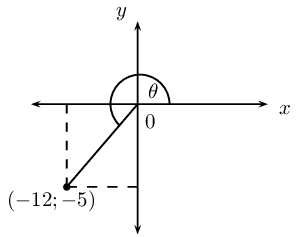Determine without using a calculator $$\sin \theta$$ and $$\cos (\text{180}\text{°} + \theta)$$.

\begin{align*} OP &= \sqrt{(-12)^2 + (-5)^2} \\ &= \sqrt{144 + 25} \\ &= \sqrt{169}\\ &= 13\\ \sin \theta &= - \frac{5}{13}\\ \cos (\text{180}\text{°} + \theta) &= - \cos \theta \\ &= - (-\frac{12}{13}) \\ &= \frac{12}{13} \end{align*}

Use a calculator to find $$\theta$$ (correct to two decimal places).

\begin{align*} \tan \theta &= \frac{5}{12} \\ \theta &= \text{180}\text{°} + \text{22,62}\text{°}\\ &= \text{202,62}\text{°} \end{align*}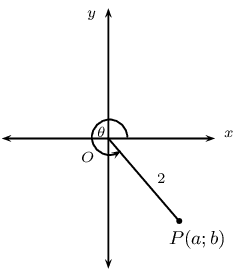In the figure, $$P$$ is a point on the Cartesian plane such that $$OP = 2$$ units and $$\theta = \text{300}\text{°}$$. Without the use of a calculator, determine:

the values of $$a$$ and $$b$$

\begin{align*} \cos \text{300}\text{°} &= \cos \text{60}\text{°} \\ & = \frac{1}{2}\\ \therefore a &= 1 \\ b &= - \sqrt{4-1} \\ & = -\sqrt{3} \end{align*}

the value of $$\sin (\text{180}\text{°} - \theta)$$

\begin{align*} \sin (\text{180}\text{°} - \theta) &= \sin \theta \\ &= \sin \text{300}\text{°} \\ &= \sin (\text{360}\text{°} - \text{60}\text{°})\\ &= \sin (-\text{60}\text{°})\\ &= -\sin \text{60}\text{°}\\ &= - \frac{\sqrt{3}}{2} \end{align*}

Solve for $$x$$ with $$x \in [-\text{180}\text{°};\text{180}\text{°}]$$ (correct to one decimal place):

$$2 \sin \frac{x}{2} = \text{0,86}$$
\begin{align*} \sin \frac{1}{2}x &= \text{0,43} \\ \frac{1}{2}x &= \text{25,467}\text{°}\\ x &= \text{50,9}\text{°} \\ \text{Or } \quad \frac{1}{2}x &=\text{180}\text{°} - \text{25,467}\text{°}\\ x &= \text{309,1}\text{°} \end{align*}
$$\tan (x + \text{10}\text{°}) = \cos \text{202,6}\text{°}$$
\begin{align*} \tan (x + \text{10}\text{°}) &= -\text{0,92}\\ x + \text{10}\text{°} &= \text{180}\text{°} -\text{42,7}\text{°}\\ &= \text{137,3}\text{°}\\ x &= \text{127,3}\text{°}\\ \text{Or} \quad x + \text{10}\text{°} &= \text{360}\text{°} - \text{42,7}\text{°}\\ &= \text{317,3}\text{°}\\ \therefore x &= \text{307,3}\text{°} \end{align*}
$$\cos^2x - 4 \sin^2x = 0$$
\begin{align*} \cos x - 2\sin x &= 0\\ \cos x &= 2\sin x\\ \frac{\sin x}{\cos x} &= \frac{1}{2}\\ \tan x &= \frac{1}{2}\\ x &= \text{26,6}\text{°}\\ \text{or } x &= -\text{180}\text{°} + \text{26,6}\text{°} \\ &= \text{206,6}\text{°}\\ \text{Or } \quad \cos x + 2\sin x &= 0\\ \cos x &= -2 \sin x\\ \frac{\sin x}{\cos x} &= -\frac{1}{2}\\ \tan x &= -\frac{1}{2}\\ x &= \text{180}\text{°} - \text{26,6}\text{°}\\ &= \text{153,4}\text{°}\\ \text{or } x &= \text{360}\text{°} - \text{26,6}\text{°} \\ &= \text{333,4}\text{°} \end{align*}

Find the general solution for the following equations:

$$\frac{1}{2} \sin (x - \text{25}\text{°}) = \text{0,25}$$
\begin{align*} \sin(x - \text{25}\text{°}) &= 0.5\\ x - \text{25}\text{°} &= \text{30}\text{°} \\ \text{ or } x - \text{25}\text{°} &= \text{180}\text{°} - \text{30}\text{°}\\ x &= \text{55}\text{°} + \text{360}\text{°}n, n \in \mathbb{Z} \\ \text{ or } x &= \text{175}\text{°} + \text{360}\text{°}n, n \in \mathbb{Z} \end{align*}
$$\sin^2x + 2 \cos x = -2$$
\begin{align*} \sin^2 x + 2\cos x + 2 &= 0\\ 1 - \cos^2 x + 2\cos x + 2 &= 0\\ - \cos^2 x + 2\cos x + 3 &= 0\\ \cos^2 x - 2\cos x - 3 &= 0\\ (\cos x - 3)(\cos x + 1) &= 0\\ \cos x &= 3 \\ & \text{no solution} \\ \text{OR} \cos x &= -1\\ x &= \text{180}\text{°} + \text{360}\text{°}n, n \in \mathbb{Z} \end{align*}

Given the equation: $$\sin 2 \alpha = \text{0,84}$$

Find the general solution of the equation.
\begin{align*} \sin 2\alpha &= \text{0,84}\\ 2\alpha &= \text{57,14}\text{°} + \text{360}\text{°}n, n \in \mathbb{Z}\\ \alpha &= \text{28,6}\text{°} + \text{180}\text{°}n\\ \text{Or } 2\alpha &= \text{180}\text{°} - \text{57,14}\text{°} + \text{360}\text{°}n, n \in \mathbb{Z}\\ 2\alpha &= \text{122,86}\text{°} + \text{360}\text{°}n\\ \alpha &= \text{61,43}\text{°} + \text{180}\text{°}n \end{align*}
Illustrate how this equation could be solved graphically for $$\alpha \in [\text{0}\text{°}; \text{360}\text{°}]$$.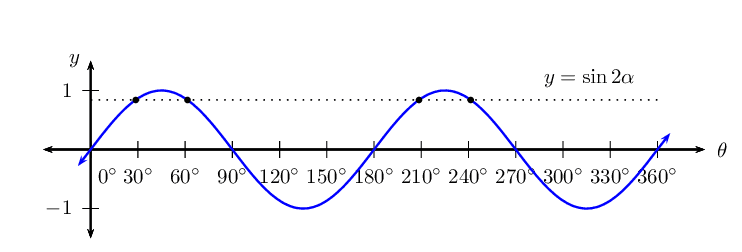Write down the solutions for $$\sin 2 \alpha = \text{0,84}$$ for $$\alpha \in [\text{0}\text{°}; \text{360}\text{°}]$$.
$$\text{28,6}\text{°}; \text{61,4}\text{°}; \text{208,6}\text{°}; \text{241,4}\text{°}$$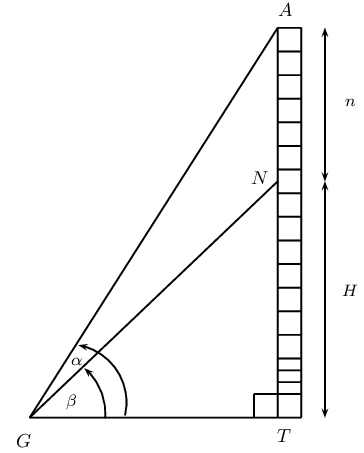$$A$$ is the highest point of a vertical tower $$AT$$. At point $$N$$ on the tower, $$n$$ metres from the top of the tower, a bird has made its nest. The angle of inclination from $$G$$ to point $$A$$ is $$\alpha$$ and the angle of inclination from $$G$$ to point $$N$$ is $$\beta$$.

Express $$A\hat{G}N$$ in terms of $$\alpha$$ and $$\beta$$.

\begin{align*} A\hat{G}N &= \alpha - \beta \end{align*}

Express $$\hat{A}$$ in terms of $$\alpha$$ and/or $$\beta$$.

\begin{align*} \hat{A} &= \text{90}\text{°} - \alpha \end{align*}

Show that the height of the nest from the ground ($$H$$) can be determined by the formula $H = \frac{n \cos \alpha \sin \beta}{\sin(\alpha - \beta)}$

\begin{align*} \text{In } \triangle GNT, & \\ \frac{H}{GN} &= \sin \beta \\ \therefore H &= GN \sin \beta \\ \text{In } \triangle AGN, & \\ \frac{n}{\sin(\alpha - \beta)} &= \frac{GN}{\sin(\text{90}\text{°} - \alpha)}\\ \therefore GN &= \frac{n \sin(\text{90}\text{°} - \alpha)}{\sin(\alpha - \beta)} \\ & = \frac{n \cos \alpha}{\sin(\alpha - \beta)} \\ \text{Substitute for } GN, & \\ H &= \frac{n \cos \alpha \sin \beta}{\sin(\alpha-\beta)} \end{align*}

Calculate the height of the nest $$H$$ if $$n = \text{10}\text{ m}$$, $$\alpha = \text{68}\text{°}$$ and $$\beta = \text{40}\text{°}$$ (give your answer correct to the nearest metre).

\begin{align*} H &= \frac{n \cos \alpha \sin \beta}{\sin(\alpha-\beta)}\\ &= \frac{10 \cos \text{68}\text{°} \sin \text{40}\text{°}}{\sin \text{28}\text{°}}\\ &= \text{5,1}\text{ m} \end{align*}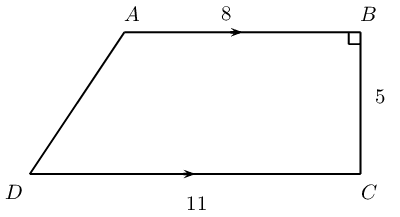Mr. Collins wants to pave his trapezium-shaped backyard, $$ABCD$$. $$AB \parallel DC$$ and $$\hat{B} = \text{90}\text{°}$$. $$DC = \text{11}\text{ m}$$, $$AB = \text{8}\text{ m}$$ and $$BC = \text{5}\text{ m}$$.

Calculate the length of the diagonal $$AC$$.
\begin{align*} AC^2 &= 8^2 + 5^2\\ &= 64 + 25 = 89\\ AC &= \text{9,43}\text{ m} \end{align*}
Calculate the length of the side $$AD$$.
\begin{align*} \text{In } \triangle ABC, &\\ \tan B\hat{A}C &= \frac{5}{8}\\ \therefore B\hat{A}C &= \text{32}\text{°}\\ A\hat{C}D = B\hat{A}C &= \text{32}\text{°} (AB \parallel DC)\\ \text{In } \triangle ADC, &\\ AD^2 &= AC^2 + DC^2 - 2.AC.AD.\cos(A\hat{C}D)\\ &= (\text{9,4})^2 + (\text{11})^2 - 2 (\text{9,4})(\text{11}) \cos(\text{32}\text{°})\\ &= 89 + 121 - 176\\ &= 34\\ \therefore AD &= \text{6,2}\text{ m} \end{align*}
Calculate the area of the patio using geometry.
\begin{align*} \text{Area } &= \frac{1}{2} \times (8+11) \times 5 \\ &= \text{47,5}\text{ m$^{2}$} \end{align*}
Calculate the area of the patio using trigonometry.
\begin{align*} \text{Area } ABCD &= \text{area } \triangle ABC + \text{area } \triangle ADC \\ &= \frac{1}{2} (8) (5) + \frac{1}{2} (9.43) (11) \sin \text{32}\text{°}\\ &= 20 + \text{27,5}\\ &= \text{47,5}\text{ m$^{2}$} \end{align*}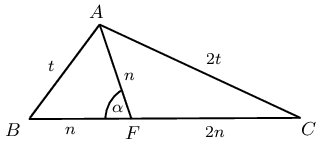In $$\triangle ABC$$, $$AC = 2A$$, $$AF = BF$$, $$A\hat{F}B = \alpha$$ and $$FC = 2AF$$. Prove that $$\cos \alpha = \frac{1}{4}$$.

\begin{align*} \text{In } \triangle ABF & \\ t^2 &= n^2 + n^2 - 2n^2 \cos \alpha \\ t^2 &= 2n^2(1 - \cos \alpha) \\ 1 - \cos \alpha &= \frac{t^2}{2n^2}\\ \cos \alpha &= 1 - \frac{t^2}{2n^2} \\ \text{In } \triangle AFC & \\ (2t)^2 &= n^2 + (2n)^2 - 4n^2 \cos (A\hat{F}C)\\ 4t^2 &= n^2 + 4n^2 - 4n^2 \cos (\text{180}\text{°} - \alpha) \\ &= 5n^2 + 4n^2 \cos \alpha\\ t^2 &= \frac{5}{4} n^2 + n^2 \cos \alpha \\ \text{Substitute for } t^2 & \\ \cos \alpha &= 1 - \frac{\frac{5}{4}n^2 + n^2 \cos \alpha}{2n^2}\\ &= \frac{2n^2 - \frac{5}{4}n^2 - n^2 \cos \alpha}{2 n^2}\\ &= \frac{\frac{3}{4}n^2 - n^2 \cos \alpha}{2n^2}\\ &= \frac{3}{8} - \frac{\cos \alpha}{2}\\ \frac{3}{2} \cos \alpha &= \frac{3}{8}\\ \therefore \cos \alpha &= \frac{1}{4} \end{align*}